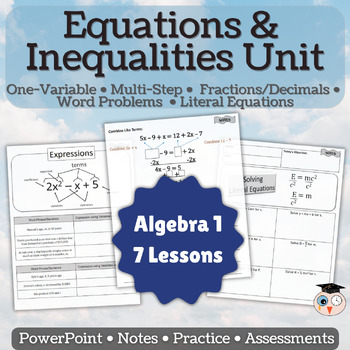# Solving Linear Equations and Inequalities Unit;
8th - 10th, Homeschool
Subjects
Resource Type
Formats Included
• Zip
•Google Apps™
\$19.50
List Price:
\$29.00
You Save:
\$9.50
Bundle
\$19.50
List Price:
\$29.00
You Save:
\$9.50
Bundle
Report this resource to TPTThis bundle contains one or more resources with Google apps (e.g. docs, slides, etc.).
Easel Activities Included
Some resources in this bundle include ready-to-use interactive activities that students can complete on any device.  Easel by TPT is free to use! Learn more.
Easel Assessment Included
Some resources in this bundle include self-grading quizzes that students can complete on any device. Easel by TPT is free to use! Learn more.

#### Products in this Bundle (7)

showing 1-5 of 7 products

#### Bonus

Unit Lesson Plan

### Description

Expressions, equations, inequalities and literal equations are key to success in Algebra and college readiness. This unit is a bundle of these concepts and will require very little time for the teacher to prepare since everything is provided for you. Each lesson contains a PowerPoint to display while students are taking notes on the PDF that is provided. Included in each lesson are practice pages and a quiz. There are digital pieces scattered throughout. All lessons have a lesson plan to guide the teacher through the PowerPoint and PDF portions.

***Be sure to download the lesson plan for the unit in the bonus material below.

Take a look at what is in each of the 7 resources:

• Expressions - Expressions are the foundation of Algebra and a great way to start your year. Students need to understand how to write expressions from key phrases, simplify expressions and evaluate expressions. (Contains 5 pages of notes, work, and quiz, 1 digital resource, 15 page PowerPoint.)
• Solving Multi-step Equations - Solving multi-step equations is a key component of high school math. Students need to know how to solve equations involving variables on both sides of the equations, the distributive property and combining like terms. This activity walks students through these problems and helps students to understand not only how to solve, but also how to check their answers. (Contains 9 pages of notes, work, and quiz, 3 digital activities, 14 page PowerPoint.)
• Solving Special Equations - The two main focuses of this lesson is to teach students to clear decimals and fractions and to recognize equations that have no solution or infinite solutions. (Contains 5 pages of notes, work, and quiz, 1 video activity, 6 page PowerPoint.)
• Writing and Solving Equations - (Contains 4 pages of notes, work, and quiz, 8 page PowerPoint.) The three main focuses of this lesson is to teach students to set up equations from phrases such as:
• Two less than three times a number is 12
• 56% of what number is 200
• Find two consecutive even integers with a sum of 34

• Equations Assessment - This resource has a combination of several assessments in digital and printable forms. You will find an array of question types with a mix of some basic math thrown in here and there. (Contains 3 digital assessments and 3 printable assessments.)
• Inequalities - The focus of this lesson is to teach students to write, solve, graph and check inequalities in one variable. (Contains 9 pages of notes, work, and quiz, 15 page PowerPoint.)
• Literal Equations - The focus of this lesson is to teach students to solve literal equations and mathematic and scientific formulas. These skills are important for their future math classes and for college readiness. (Contains 3 pages of notes, work, and quiz, 5 page PowerPoint.)
Total Pages